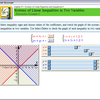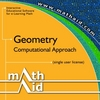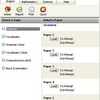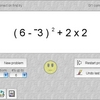# Mathaid sat. math practice

Highly interactive tutorials and self-test system for individual e-learning, home schooling, college and high school computer learning centers, and distance learning. The product emphasizes on building problem-solving skills. Tutorials include the reviews of basic concepts, interactive examples, and standard problems with randomly generated parameters. The self-test system allows selecting topics and length for a test, saving test results, and getting the test review. Topics covered: factors and multiples, fractions, mixed numbers, ratios and proportions, percent, exponents and radicals, operations with polynomials, factoring polynomials, roots of polynomial equations, rational expressions, solving linear equations, solving linear inequalities, solving equations involving absolute values, solving inequalities involving absolute values, the distance formula, the midpoint formula, slope of a line, equations of straight lines, functions and graphs, linear functions, systems of two linear

## Related software (5)## MathAid Algebra II

Tutorial contains basic concepts, interactive examples, and problems with randomly generated parameters. A customer is allowed to select chapters for a test, get the test review, ...## MathAid Geometry

Tutorial contains basic concepts, interactive examples, and problems with randomly generated parameters. A customer is allowed to select chapters for a test, get the test review, ...## Primary 4 Test Papers

Primary 4 English, Maths & Science test papers. 2500 challenging quizzes to practice. Questions are modelled closely to primary education curriculum. All test papers comes with mo ...## Smooth Operators

Smooth Operators is a complete solution for learning, practicing, and testing the order of operations. An interactive lesson teaches concepts. Practice by clicking operands, or tes ...## Geometry Quiz

Challenge your mind and try to solve all equations.